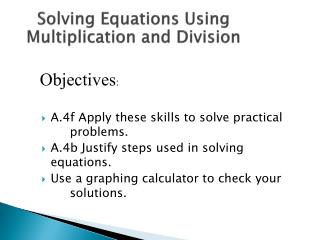DownloadDownload PresentationA.4f Apply these skills to solve practical problems.

# A.4f Apply these skills to solve practical problems.

Download Presentation## A.4f Apply these skills to solve practical problems.

- - - - - - - - - - - - - - - - - - - - - - - - - - - E N D - - - - - - - - - - - - - - - - - - - - - - - - - - -
##### Presentation Transcript

1. Solving Equations Using Multiplication and Division Objectives: • A.4f Apply these skills to solve practical problems. • A.4b Justify steps used in solving equations. • Use a graphing calculator to check your solutions.

2. Remember, To Solve an Equation means... • To isolate the variable on one side of the equation. • Ex: x = 5 is solved for x. • y = 2x - 1 is solved for y.

3. Multiplication Property of Equality • For any numbers a, b, and c, if a = b, then ac= bc. What it means: You can multiply BOTH sides of an equation by any number and the equation will still hold true.

4. An easy example: • Would you ever put deodorant under just one arm? • Would you ever put nail polish on just one hand? • Would you ever wear just one sock? We all know that 3 = 3. Does 3(4) = 3? NO! But 3(4) = 3(4). The equation is still true if we multiply both sides by 4.

5. Let’s try another example! • Always check your solution!! • The original problem is x = 4 2 • Using the solution x = 8, Does 8/2 = 4? • YES! 4 = 4 and our solution is correct. x = 4 2 Multiply each side by 2. (2)x = 4(2) 2 x = 8

6. Division Property of Equality For any numbers a, b, and c (c≠0), if a = b, then a/c= b/c What it means: • You can divide BOTH sides of an equation by any number, except zero, and the equation will still hold true. • Why did we add c ≠ 0?

7. 2 Examples: 1) 4x = 24 Divide both sides by 4. 4x = 24 4 4 x = 6 • Does 4(6) = 24? YES! 2) -6y = 18 Divide both sides by -6. -6y = 18 -6 -6 y = -3 • Does -6(-3) = 18? YES!

8. A fraction times a variable: The two step method: Ex: 2x = 4 3 1. Multiply by 3. (3)2x = 4(3) 3 2x = 12 2. Divide by 2. 2x = 12 2 2 x = 6 The one step method: Ex: 2x = 4 3 1. Multiply by the reciprocal. 32x = 4 3 2 3 2 x = 6

9. Answer Now Solve -3v = -129 • v = -126 • v = -43 • v = 43 • v = 126

10. Answer Now Which step clears the fraction in • Multiply by 3 • Multiply by 5 • Multiply by -12 • Multiply by -5

11. Answer Now Solve • b = -56 • b = -14 • b = 14 • b = 56

12. Try these on your own...